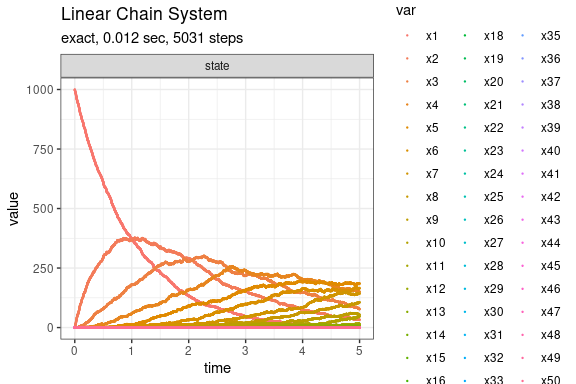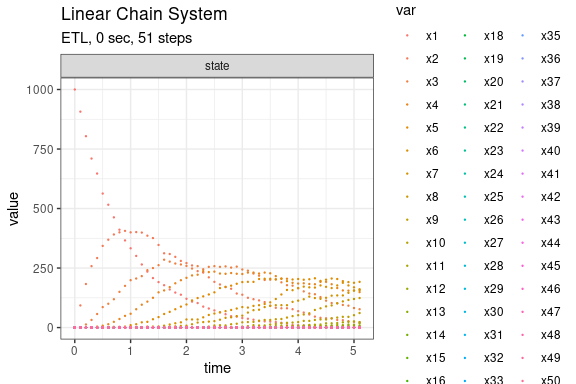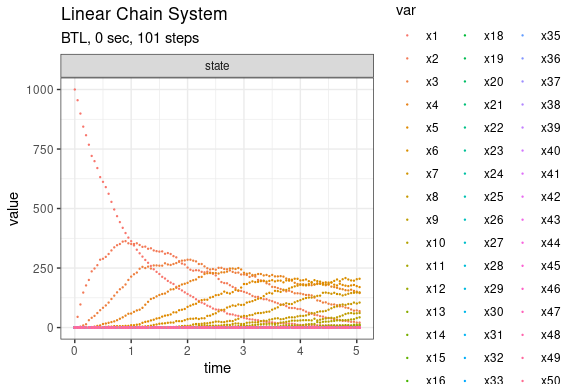# Linear Chain System (Cao et al., 2004)

The Linear Chain System consists of M chain reactions with M+1 species as follows:

  S_1 --c1--> S_2
S_2 --c2--> S_3
...
S_M --cM--> S_(M+1)

Define parameters

library(GillespieSSA2)
sim_name <- "Linear Chain System"
M <- 50
params <- c(c = 1)
final_time <- 5
initial_state <- c(1000, rep(0, M))
names(initial_state) <- paste0("x", seq_len(M+1))

Define the reactions

reactions <- lapply(
seq_len(M),
function(i) {
effect <- c(-1, 1)
names(effect) <- paste0("x", c(i, i + 1))

reaction(paste0("c * x", i), effect)
}
)

Run simulations with the Exact method

set.seed(1)
out <- ssa(
initial_state = initial_state,
reactions = reactions,
params = params,
final_time = final_time,
method = ssa_exact(),
sim_name = sim_name
)
plot_ssa(out)Run simulations with the Explict tau-leap method

set.seed(1)
out <- ssa(
initial_state = initial_state,
reactions = reactions,
params = params,
final_time = final_time,
method = ssa_etl(tau = .1),
sim_name = sim_name
)
plot_ssa(out)Run simulations with the Binomial tau-leap method

set.seed(1)
out <- ssa(
initial_state = initial_state,
reactions = reactions,
params = params,
final_time = final_time,
method = ssa_btl(mean_firings = 50),
sim_name = sim_name
)
plot_ssa(out)